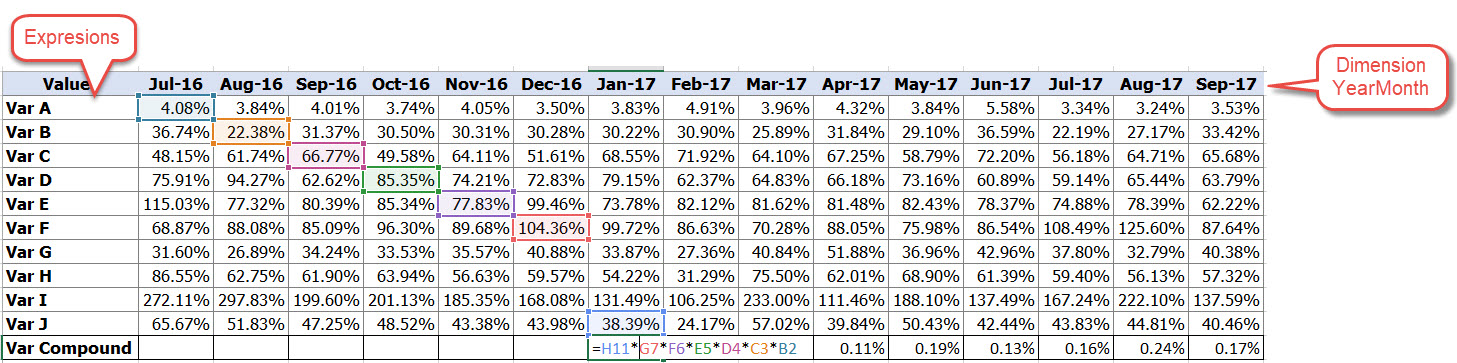QlikView Creating Analytics

Discussion Board for collaboration related to Creating Analytics for QlikView.

New Contributor

dynamic sum crossing year month dimension

Hi Everyone,

I would like to make pivot chart with Dimension YearMonth and 11 expression, I got problem to calculate my last expression, is there anyway to calculate my last expression, how to represent the formula into set analysis?

Var Compound=Sum Var J(YearMonth) * sum Var F(YearMonth)-1 * Sum Var E(YearMonth)-2 * Sum Var D(YearMonth)-3 * Sum Var C(YearMonth)-4 * Sum Var B(YearMonth)-5 * Sum Var A(YearMonth)-6I have tried to write set expression like below, but it does't work like expected. Has anyone have suggestion?

=

//Var J

(Sum({<[Fact_Type]={'FLOWRATE'},[Code]={'amount'} >} FR_AmountWO)

/(sum({<[Fact_Type]={'FLOWRATE'},[Code]={'amount'} >} FR_Amount_151_180_b )

+ sum({<[Fact_Type]={'FLOWRATE'},[Code]={'amount'} >} FR_Amount_180_b ))

)

*

//Var F

sum({<YeartoMonth ={"\$(=Date(addmonths(Max(YeartoMonth), -1), 'YYYYMM'))"}, [Fact_Type]={'FLOWRATE'},[Code]={'amount'}>} FR_Amount_151_180 )

/sum({<YeartoMonth ={"\$(=Date(addmonths(Max(YeartoMonth), -1), 'YYYYMM'))"}, [Fact_Type]={'FLOWRATE'},[Code]={'amount'}>} FR_Amount_121_150_b )

*

//Var E

(sum({<YeartoMonth ={">=\$(=Date(addmonths(Max(YeartoMonth), -2), 'YYYYMM'))"}, [Fact_Type]={'FLOWRATE'},[Code]={'amount'}>} FR_Amount_121_150 )/

sum({<YeartoMonth ={">=\$(=Date(addmonths(Max(YeartoMonth), -2), 'YYYYMM'))"}, [Fact_Type]={'FLOWRATE'},[Code]={'amount'}>} FR_Amount_91_120_b )

)

*

//Var D

sum({<YeartoMonth ={"=\$(=Date(addmonths(Max(YeartoMonth), -3), 'YYYYMM'))"}, [Fact_Type]={'FLOWRATE'},[Code]={'amount'}>} FR_Amount_91_120 )

/sum({<YeartoMonth ={"=\$(=Date(addmonths(Max(YeartoMonth), -3), 'YYYYMM'))"}, [Fact_Type]={'FLOWRATE'},[Code]={'amount'}>} FR_Amount_61_90_b )

*

//Var C

sum({<YeartoMonth ={"=\$(=Date(addmonths(Max(YeartoMonth), -4), 'YYYYMM'))"}, [Fact_Type]={'FLOWRATE'},[Code]={'amount'}>} FR_Amount_61_90 )

/sum({<YeartoMonth ={"=\$(=Date(addmonths(Max(YeartoMonth), -4), 'YYYYMM'))"}, [Fact_Type]={'FLOWRATE'},[Code]={'amount'}>} FR_Amount_31_60_b )

*

//Var B

sum({<YeartoMonth ={"=\$(=Date(addmonths(Max(YeartoMonth), -5), 'YYYYMM'))"}, [Fact_Type]={'FLOWRATE'},[Code]={'amount'}>} FR_Amount_31_60 )

/sum({<YeartoMonth ={"=\$(=Date(addmonths(Max(YeartoMonth), -5), 'YYYYMM'))"}, [Fact_Type]={'FLOWRATE'},[Code]={'amount'}>} FR_Amount_1_30_b )

*

//Var A

=sum({<YeartoMonth ={"=\$(=Date(addmonths(Max(YeartoMonth), -6), 'YYYYMM'))"}, [Fact_Type]={'FLOWRATE'},[Code]={'amount'}>} FR_Amount_1_30 )

/sum({<YeartoMonth ={"=\$(=Date(addmonths(Max(YeartoMonth), -6), 'YYYYMM'))"}, [Fact_Type]={'FLOWRATE'},

={'amount'}>} FR_AmountCurrent_b)

Tags (1)